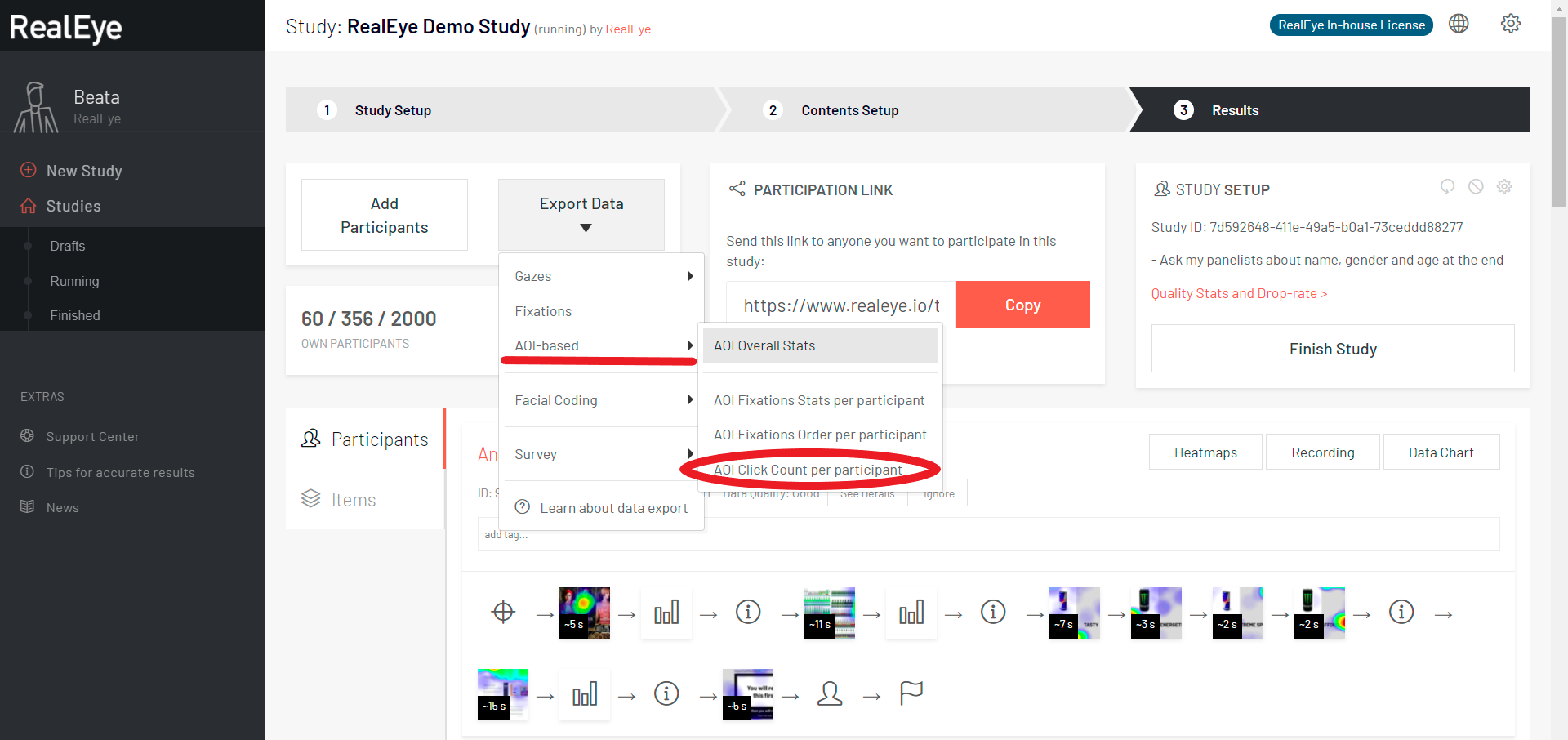# CSV file: AOI click countThe "AOI click count" CSV contains the following fields:

• study_id - UUID,
• item_id - UUID,
• item_filename - the name of your file, as it was named while adding to study,
• tester_id - UUID,
• tester_external_id - (UUID) in case if you provide one by connecting the study with an external tool, i.e. a survey,
• tester_display_name - the name that a tester gives while asked about a name, gender and age at the end of the study (if you have chosen that option), i.e. "Anna, Female, 27",
• 1 = very low,
• 2 = low,
• 3 = average,
• 4 = good,
• 5 = very good,
• 6 = perfect;
• 1 = very low,
• 2 = low,
• 3 = average,
• 4 = good,
• 5 = very good,
• 6 = perfect;
• test_item_display_order - a number indicating the order in which the items were displayed,
• aoi_id - UUID,
• aoi_name - the name that you give to an AOI while saving it,
• aoi_size_percents - expressed as the percentage value relative to the size of the entire image,
• aoi_time_range_from_ms - the start time from which the values of the AOI were calculated (you can set this time with the orange slider under the heatmap),
• aoi_time_range_to_ms - the end time to which the values of the AOI were calculated (you can set this time with the orange slider under the heatmap),
• aoi_click_total_count - how many clicks each participant made within this AOI,
• aoi_click_average_time_to_first_click_ms - TTFC (time to first click) within given AOI,
• aoi_fixation_ttff_ms - TTFF (time to first fixation) within given AOI.
• notes - this column contains:
• RealEye version,
• fixation filter settings:
• gazepoint interpolation (Hz) - to improve calculations we interpolate the data to 60 Hz;
• gazepoint interpolation max gap (ms) - we interpolate data if the timestamp gap between two input values is not bigger than this value (50 ms);
• gaze velocity threshold;
• min. fixation duration (Ms);
• noise reduction level.

See the CSV file for the sample study: